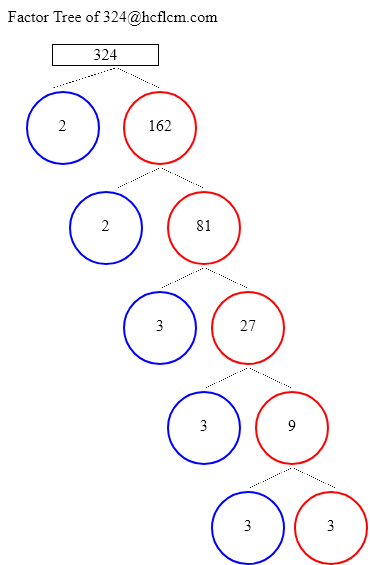# Factors of 324 | Find the Factors of 324 by Factoring Calculator

Factoring Calculator calculates the factors and factor pairs of positive integers.

Factors of 324 can be calculated quickly with the help of Factoring Calculator i.e. 1, 2, 3, 4, 6, 9, 12, 18, 27, 36, 54, 81, 108, 162, 324 positive integers that divide 324 without a remainder.

Factors of 324 are 1, 2, 3, 4, 6, 9, 12, 18, 27, 36, 54, 81, 108, 162, 324. There are 15 integers that are factors of 324. The biggest factor of 324 is 324.

Factors of:

### Factor Tree of 324 to Calculate the Factors

 324 2 162 2 81 3 27 3 9 3 3Factors of 324 are 1, 2, 3, 4, 6, 9, 12, 18, 27, 36, 54, 81, 108, 162, 324. There are 15 integers that are factors of 324. The biggest factor of 324 is 324.

Positive integers that divides 324 without a remainder are listed below.

• 1
• 2
• 3
• 4
• 6
• 9
• 12
• 18
• 27
• 36
• 54
• 81
• 108
• 162
• 324

### Factors of 324 in pairs

• 1 × 324 = 324
• 2 × 162 = 324
• 3 × 108 = 324
• 4 × 81 = 324
• 6 × 54 = 324
• 9 × 36 = 324
• 12 × 27 = 324
• 18 × 18 = 324
• 27 × 12 = 324
• 36 × 9 = 324
• 54 × 6 = 324
• 81 × 4 = 324
• 108 × 3 = 324
• 162 × 2 = 324
• 324 × 1 = 324

### Factors of 324 Table

FactorFactor Number
1one
2two
3three
4four
6six
9nine
12twelve
18eighteen
27twenty seven
36thirty six
54fifty four
81eighty one
108one hundred eight
162one hundred sixty two
324three hundred twenty four

### How to find Factors of 324?

As we know factors of 324 are all the numbers that can exactly divide the number 324 simply divide 324 by all the numbers up to 324 to see the ones that result in zero remainders. Numbers that divide without remainder are factors and in this case below are the factors

1, 2, 3, 4, 6, 9, 12, 18, 27, 36, 54, 81, 108, 162, 324 are the factors and all of them can exactly divide number 324.

### Frequently Asked Questions on Factors of 324

1. What are the factors of 324?

Answer: Factors of 324 are the numbers that leave a remainder zero. The ones that can divide 324 exactly i.e. factors are 1, 2, 3, 4, 6, 9, 12, 18, 27, 36, 54, 81, 108, 162, 324.

2.What are Factor Pairs of 324?

Answer:Factor Pairs of 324 are

• 1 × 324 = 324
• 2 × 162 = 324
• 3 × 108 = 324
• 4 × 81 = 324
• 6 × 54 = 324
• 9 × 36 = 324
• 12 × 27 = 324
• 18 × 18 = 324
• 27 × 12 = 324
• 36 × 9 = 324
• 54 × 6 = 324
• 81 × 4 = 324
• 108 × 3 = 324
• 162 × 2 = 324
• 324 × 1 = 324

3. What is meant by Factor Pairs?

Answer:Factor Pairs are numbers that when multiplied together will result in a given product.